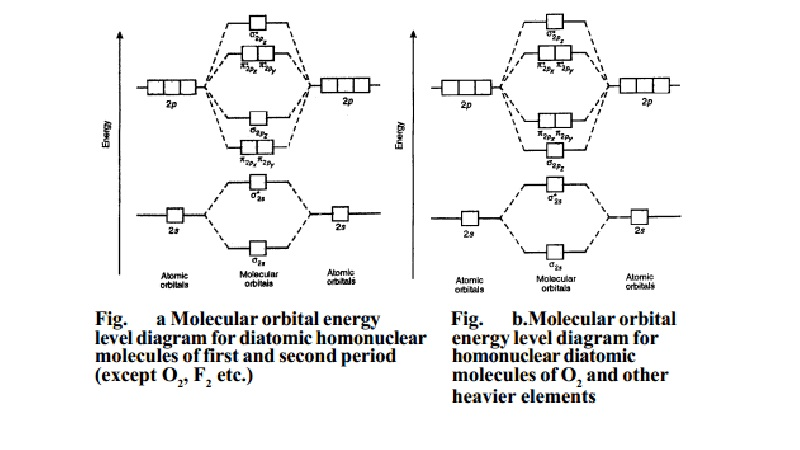Home | | Chemistry | | Chemistry | Molecular Orbital Theory: Energy level diagram for molecular orbitals

# Molecular Orbital Theory: Energy level diagram for molecular orbitalsMolecular orbital theory was put forward by Hund and Mullikan in 1932. This theory is modern and more rational. This theory assume that in molecules, atomic orbitals lose their identity and the electrons in molecules are present in new orbitals called molecular orbitals. A brief outline of this theory is given below:

Molecular Orbital Theory : Energy level diagram for molecular orbitals

MOLECULAR ORBITAL THEORY

Molecular orbital theory was put forward by Hund and Mullikan in 1932.

This theory is modern and more rational. This theory assume that in molecules, atomic orbitals lose their identity and the electrons in molecules are present in new orbitals called molecular orbitals. A brief outline of this theory is given below:

(i) In a molecule, electrons are present in new orbitals called molecular orbitals. (ii) Molecular orbitals are formed by combination of atomic orbitals of equal energies (in case of homonuclear molecules) or of comparable energies (in case of heteronuclear molecules).

(iii) The number of molecular orbitals formed is equal to the number of atomic orbitals undergoing combination.

(iv) Two atomic orbitals can combine to form two molecular orbitals. One of

these two molecular orbitals one has a lower energy and the other has a higher energy. The molecular orbital with lower energy is called bonding molecular orbital and the other with higher energy is called anti bonding molecular orbital.

(v) The shapes of molecular orbitals depend upon the shapes of combining atomic orbitals.

(vi) The bonding molecular orbitals are represented by s (sigma), p (pi), d (delta) and the antibonding molecular orbitals are represented by s*, p*, d*.

(vii) The molecular orbitals are filled in the increasing order of their energies,  starting with orbital of least energy. (Aufbau principle).

(viii) A molecular orbital can accommodate only two electrons and these two electrons must have opposite spins. (Paul's exclusion principle).

(ix) While filling molecular orbitals of equal energy, pairing of electrons does not take place until all such molecular orbitals are singly filled with electrons having parallel spins. (Hund's rule).

Energy level diagram for molecular orbitals

In case of homonuclear diatomic molecules, combination of two 1s atomic orbitals of participating atoms give rise to two new molecular orbitals designated as s1s and s*1s. In the same manner the 2s and three 2p-orbitals of each atom i.e., eight atomic orbitals can give rise to eight new molecular orbitals viz.,

s2s , s2s , P 2px , P *2px , P 2py , P *2py , s2pz , s2pz .

Atomic Structure and Chemical Bonding

Energy levels of these molecular orbitals have been determined experimentally by spectroscopic studies.The order of increasing energy in case of the diatomic homonuclear molecules of first and second period of the periodic table is as given below:

s1s < s*1s < s2s < s*2s < P2px = P 2py < s2pz < P *2px = P *2py < s*2pz

This order of energies of various molecular orbitals is valid for molecules or ions like, H2, H2+, He2+, He2 (hypothetical), Li2, Be2(hypothetical), B2, C2 and N2molecules. This energy diagram for the molecular orbitals is shown in Fig.1 However, experimental evidence for oxygen and heavier diatomic molecules have  shown that above sequence of energy levels of MOs is not correct. In case of  these elements, the order of energy levels of s2pz , P 2pxand P 2py is reversed i.e., s2pz has lesser energy than P 2px or P 2py . Thus, the order of increasing energy of  MOs for these molecules is as follows.

s1s < s*1s < s2s < s*2s < s 2pz < P 2px = P 2py < P *2px = P *2py < s*2pz 1s

This order of energies of various MOs is valid for molecules or ions like O2, O (super oxide ion), O22-(peroxide ion), F2 and Ne2 (hypothetical). This energy level diagram for MOs is shown in Fig. (b).

Study Material, Lecturing Notes, Assignment, Reference, Wiki description explanation, brief detail

Related Topics